# Use two phases to cut current and improve EMI

## Linear Technology LTC3862

In dc/dc-converter applications in which the input voltage may be lower or higher than the output voltage, you can use either a flyback converter or a SEPIC (single-ended-primary-inductor converter). SEPICs offer lower input-current ripple and higher efficiency than do flyback designs. Both converters suffer from relatively high output-current ripple, especially at high load currents and low input voltages. As output-current ripple increases, so does the circuit's output-filter-capacitance requirement, which increases size and cost. You can reduce output-current and -voltage ripple without increasing the application size and cost by using a multiphase SEPIC or flyback converter. Using a multiphase flyback circuit also greatly reduces the input-current ripple.

To evaluate the benefits of a dual-phase versus a single-phase SEPIC, this Design Idea compares two designs running at 300-kHz switching frequency. For consistency, both examples use the same power components, resulting in twice the output power in the two-phase design.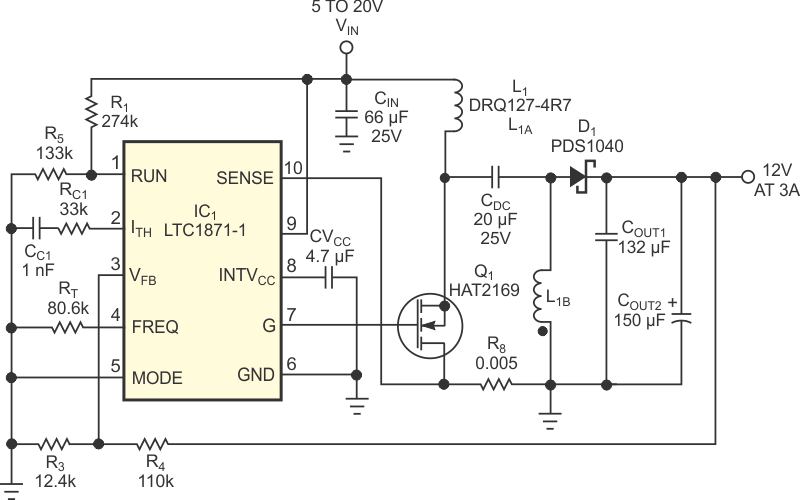Figure 1. A single-phase SEPIC has low input ripple currents and is suitable for power levels of 5 to 50 W.

The single-phase SEPIC circuit can generate 3 A of output current (Figure 1). SEPICs are typically 1 to 2% more efficient than flyback converters. Figure 2 shows the output diode's current (bottom trace) at minimum input voltage and maximum load and the output-voltage ripple (top trace). The circuit's output capacitors must handle the peak output-diode current of 14 A. Even though the circuit uses four low ESR (equivalent-series-resistance) output capacitors, output-voltage ripple is still 110 mV p-p. The aluminum output capacitor, COUT2, doesn't help much in reducing the output ripple due to its much higher ESR. COUT2 mainly helps reduce load transients by adding bulk capacitance to the output rail.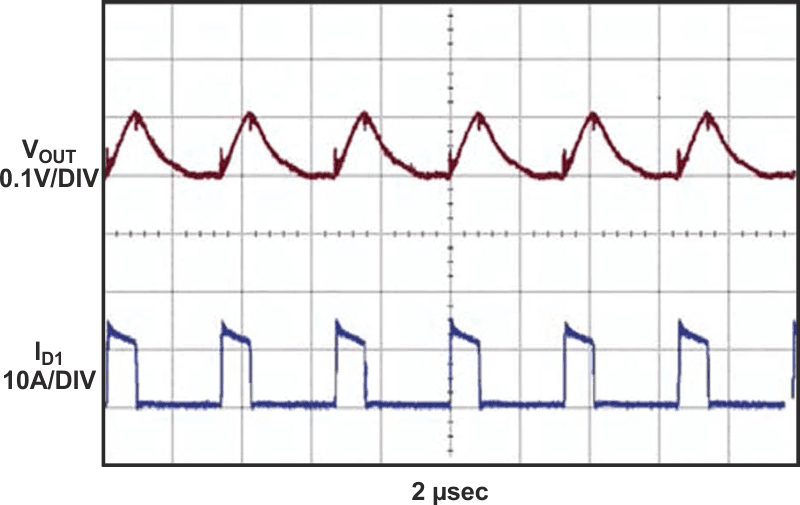Figure 2. The single-phase circuit in Figure 1 has a peak capacitor output current of about 14 A (bottom trace).

Figure 3 shows a two-phase converter, which is similar to the single-phase converter in Figure 1 except for the addition of an identical second-phase power stage. The second phase halves the peak inductor, MOSFET, and output-diode currents. The 50% lower peak output-diode currents produce 50% lower output ripple (Figure 4). Also, the output-ripple-current frequency doubles, thus making it easier to filter out with an additional LC filter if necessary.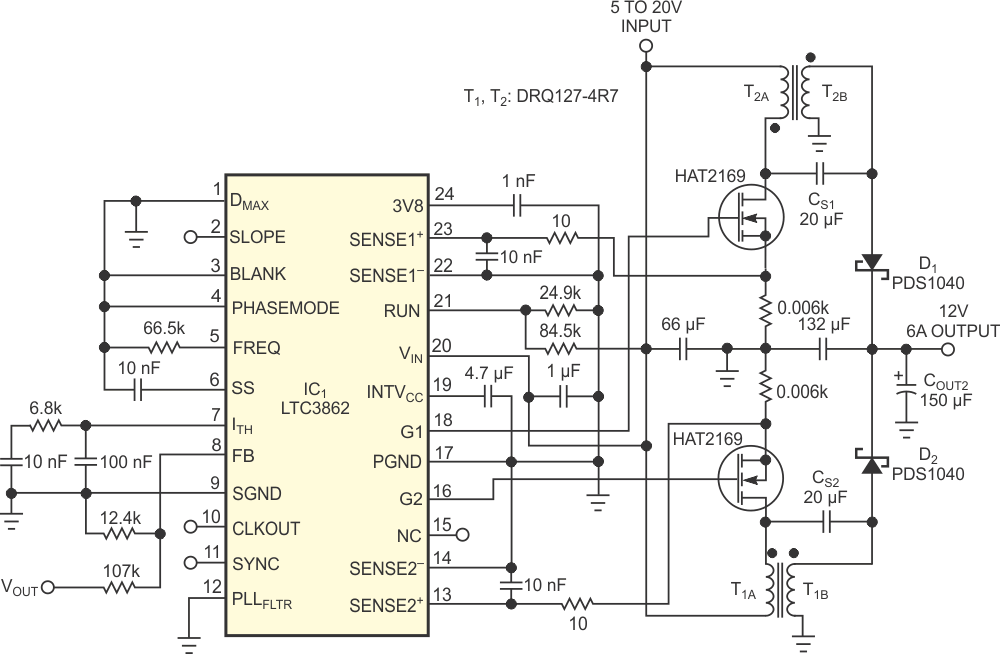Figure 3. By adding second power stage and shifting the phase by 180°, you can reduce the output ripple currents by more than 50%.

The benefits of using a dual-phase converter become clear when you consider output-capacitor ripple current (Figure 5). The two-phase converter's output-capacitor ripple current is always lower than that of an equivalent single-phase converter. Depending on the duty cycle, the two-phase converter's output-capacitor ripple current can approach 0 A at a 50% duty cycle. Inductor ripple current is still present, and you can reduce it by using larger inductors.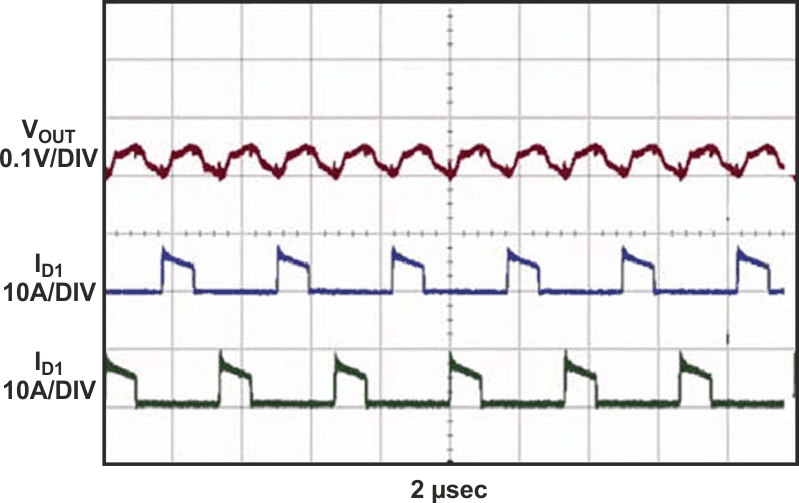Figure 4. The output ripple current is 50% lower for a two-phase SEPIC. The output ripple voltage is 50% lower than that of a single-phase design with the same output capacitors.

Using a two-phase converter means that you can use smaller inductors, MOSFETs, output diodes, and output capacitors than you can use in an equivalent single-phase converter. Because high-power designs may need to use more than one MOSFET anyway, a dual-phase design may need only one additional smaller inductor and one smaller diode. Output LC filters can also be smaller because of the doubling of the output ripple frequency. Finally, the EMI performance of a dual-phase SEPIC should be better than that of a single-phase converter because of lower current slew rates and smaller current loops.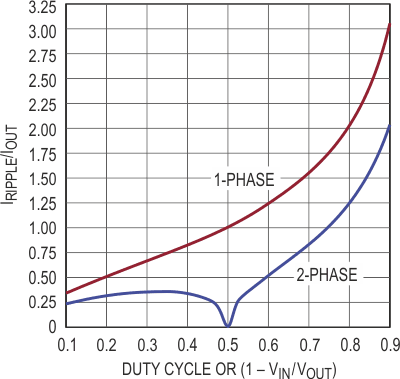Figure 5. The normalized output-capacitor ripple current for  single- and dual-phase SEPICs shows lower output ripple with a two-phase design.

## Materials on the topic

EDN